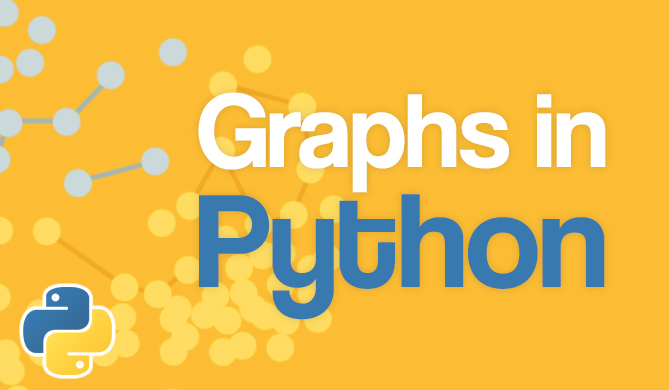Linear Search in JavaScript

# Linear Search in JavaScript### Introduction

Searching, in the context of Computer Science, is the process of locating a particular element in the given list/array. If we pay close attention, we can find search algorithms everywhere.

Consider the process of logging into a website. Entered email and password are searched against the existing key-value pairs in the database to validate the user.

In this article, let us look at the most basic algorithm to search through a given list of elements - Linear Search.

### Understanding Linear Search

The Linear Search algorithm is a set of instructions to traverse the given list and check every element in the list until we find whatever element we are looking for. The technical term for the element we are looking for is - key.

The algorithm goes from the leftmost (or starting) element and continues searching until it either finds the desired element or goes through all the elements in the list.

If the element is found, we will return the position (or index) of the element. If the element we are looking for doesn't exist in the list, we generally return -1.

### Implementation of Linear Search in JavaScript

We can traverse through the given list using a for loop. Let's look at the implementation of Linear Search:

function linearSearch(arr, key){
for(let i = 0; i < arr.length; i++){
if(arr[i] === key){
return i
}
}
return -1
}


Here we are going through all the elements in an array and comparing each element with the key. If we find a match, we return the index of the element. In our case, the variable i keeps track of where we are in the array, and if we find a match, we return the current value for i.

In case the element doesn't exist in our list, the linearSearch function won't return any i value from the loop. We just return -1 after the loop to show that the function didn't find the desired element.

### Global Linear Search

In the previous implementation, we return a value after we come across the first occurrence of the element we are looking for (key). But what if we want to the indices of all the occurrences of a given element?

That's where global linear search comes in. It is a variant of linear search where we are looking for multiple occurrences of a given element.

Let's look at the implementation of the global linear search:

function globalLinearSearch(arr, key){
let results = []
for(let i = 0; i < arr.length; i++){
if(arr[i] === key){
results.push(i)
}
}
// If results array is empty, return -1
if(!results){
return -1
}

return results
}


In this case, instead of immediately returning the index of the matching element, we are storing it in an array. In the end, we are returning the array of indices.

### The Efficiency of Linear Search

Linear Search is a classic example of a brute-force algorithm. This means that the algorithm doesn't use any logic to try and do what it's supposed to quickly, or to somehow reduce the range of elements in which it searches for key. Other search algorithms aim to do this more efficiently by some sort of preprocessing of the list/array, for example sorting it.

The time complexity of Linear Search is O(n), where n is the number of elements in the list we're searching. This is because we always consider the worst-case while calculating the time complexity. In the case of Linear Search (as with most search algorithms) the worst-case occurs when the element doesn't exist in the list. In this situation, we'd need to go through all the n elements to determine that the element isn't there.

### Conclusion

In this article, we have seen the logic behind linear search, and then using that knowledge, we implemented the algorithm in JavaScript. We have also looked at the time complexity for the linear search algorithm.

It's by far the simples search algorithm, one that doesn't use any logic to try and discard a particular range for searching or with a focus on speed.

Last Updated: October 30th, 2020

### You might also like...

Get tutorials, guides, and dev jobs in your inbox.

Abhilash KakumanuAuthor

Hey, I am a full-stack web developer located in India. I am a curious person who is always trying to wrap my head around new technologies. In my free time, I read novels and play with my dog!Free
Course

### Graphs in Python - Theory and Implementation

# python# data structures# algorithms# computer science

Graphs are an extremely versatile data structure. More so than most people realize! Graphs can be used to model practically anything, given their nature of...DetailsProject

### React State Management with Redux and Redux-Toolkit

# javascript# React

Coordinating state and keeping components in sync can be tricky. If components rely on the same data but do not communicate with each other when...Details